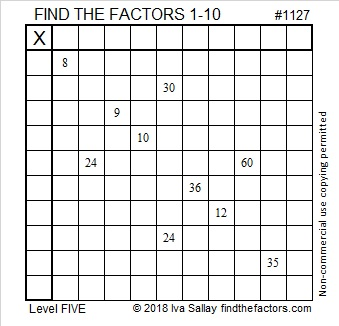# 1127 and Level 5

If the clues in this puzzle were in a Find the Factors 1 – 12, puzzle, the needed factors might be completely different than the ones in this puzzle’s solution. Fortunately, we can only use factors from 1 to 10, so this puzzle will make you think, but shouldn’t be so difficult.Print the puzzles or type the solution in this excel file: 10-factors-1121-1133

Here are a few facts about the number 1127:

• 1127 is a composite number.
• Prime factorization: 1127 = 7 × 7 × 23, which can be written 1127 = 7² × 23
• The exponents in the prime factorization are 2 and 1. Adding one to each and multiplying we get (2 + 1)(1 + 1) = 3 × 2  = 6. Therefore 1127 has exactly 6 factors.
• Factors of 1127: 1, 7, 23, 49, 161, 1127
• Factor pairs: 1127 = 1 × 1127, 7 × 161, or 23 × 49
• Taking the factor pair with the largest square number factor, we get √1127 = (√49)(√23) = 7√23 ≈ 33.570821127 is palindrome 5115 in BASE 6 because 5(6³) + 1(6²) + 1(6) + 5(1) = 1127

This site uses Akismet to reduce spam. Learn how your comment data is processed.